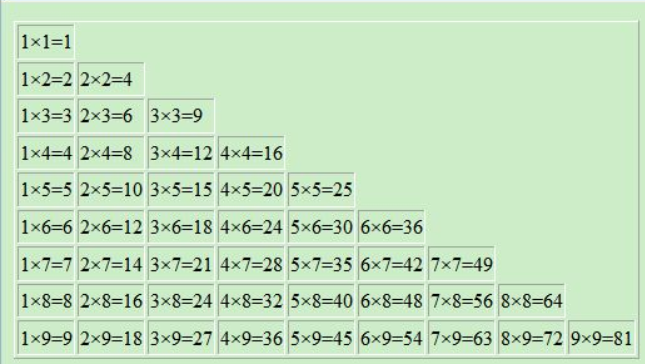# C#之打印乘法表1.我们需要打印出九行；

2.每行中最大列数等于行数；``````        public void Display1()
{
Console.WriteLine("乘法表：");
for (int i = 1; i <= 9; i++) // 循环输出乘法表行数
{
for (int j = 1; j <= i; j++) // 循环输出乘法表列数
{
Console.Write(i + "*" + j + "=" + i * j + "  ");

}
Console.WriteLine();

}
Console.WriteLine();
}
````````````        public void Display2()
{
Console.WriteLine("乘法表：");
for (int i = 1; i <= 9; i++) // 循环输出乘法表左边数值
{
for (int j = 1; j <= i; j++) // 循环输出乘法表右边数值
{
if ((i == 3 || i == 4) && j == 2) // 将乘法表排序整齐
{
Console.Write(i + "*" + j + "=" + i * j + "   ");
}
else {
Console.Write(i + "*" + j + "=" + i * j + "  ");
}

}
Console.WriteLine();

}
}
``````

code开始。